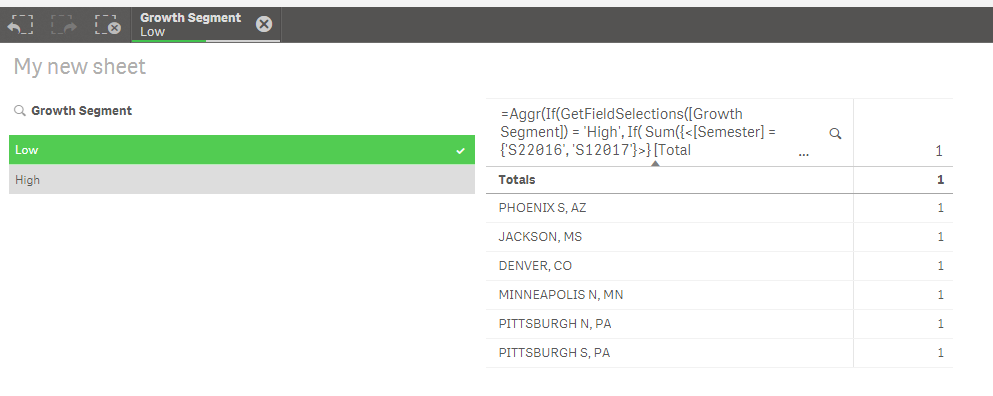# New to Qlik Sense

Discussion board where members can get started with Qlik Sense.

Announcements
Make your voice heard! Participate in the 2020 Wisdom of Crowds® Survey. BEGIN SURVEY
Highlighted
Contributor

## second part of if condition not working

Hi All,

My aim is to get territory names on field selection- 'High', if Sales growth of the territory is greater than avg sales growth and to get territory names on field selection- 'Low', if Sales growth of the territory is lower than avg sales growth. Please check the code provided below:

if(GetFieldSelections([Growth Segment])='High',

if((((AGGR(sum(if([Semester]='S22016',[Total Sales],if([Semester]='S12017',[Total Sales],0))),[Territory Name]))-

(AGGR(sum(if([Semester]='S22015',[Total Sales],if([Semester]='S12016',[Total Sales],0))),[Territory Name])))/

(AGGR(sum(if([Semester]='S22015',[Total Sales],if([Semester]='S12016',[Total Sales],0))),[Territory Name])))>

((sum(total if([Semester]='S22016',[Total Sales],if([Semester]='S12017',[Total Sales],0))))-

(sum(total if([Semester]='S22015',[Total Sales],if([Semester]='S12016',[Total Sales],0)))))/

(sum(total if([Semester]='S22015',[Total Sales],if([Semester]='S12016',[Total Sales],0)))),[Territory Name],0),

if((((Aggr(sum(if([Semester]='S22016',[Total Sales],if([Semester]='S12017',[Total Sales],0))),[Territory Name]))-

(AGGR(sum(if([Semester]='S22015',[Total Sales],if([Semester]='S12016',[Total Sales],0))),[Territory Name])))/

(AGGR(sum(if([Semester]='S22015',[Total Sales],if([Semester]='S12016',[Total Sales],0))),[Territory Name])))<

((sum(total if([Semester]='S22016',[Total Sales],if([Semester]='S12017',[Total Sales],0))))-

(sum(total if([Semester]='S22015',[Total Sales],if([Semester]='S12016',[Total Sales],0)))))/

(sum(total if([Semester]='S22015',[Total Sales],if([Semester]='S12016',[Total Sales],0)))),[Territory Name],0))

It is working fine for the field selection-'High' but not for low. Can anybody guide me where am I going wrong? Thanks.

Best Regards,

1 Solution

Accepted Solutions
HighlightedMVP

## Re: second part of if condition not working

This seems to have worked

=Aggr(If(GetFieldSelections([Growth Segment]) = 'High',

If(

Sum({<[Semester] = {'S22016', 'S12017'}>} [Total Sales])/Sum({<[Semester] = {'S22015', 'S12016'}>} [Total Sales]) >

Sum(TOTAL {<[Semester] = {'S22016', 'S12017'}>} [Total Sales])/Sum(TOTAL {<[Semester] = {'S22015', 'S12016'}>} [Total Sales]), [Territory Name]),

If(

Sum({<[Semester] = {'S22016', 'S12017'}>} [Total Sales])/Sum({<[Semester] = {'S22015', 'S12016'}>} [Total Sales]) <

Sum(TOTAL {<[Semester] = {'S22016', 'S12017'}>} [Total Sales])/Sum(TOTAL {<[Semester] = {'S22015', 'S12016'}>} [Total Sales]), [Territory Name])), [Territory Name])Here Growth Segment is a field from an Island table

22 Replies
Highlighted
Contributor

## Re: second part of if condition not working

Hi Rahul,

could you share your qvf file? Imo you should modify your script and prepare your data for this measure.

Thanks.

HighlightedPartner

## Re: second part of if condition not working

What are you trying to do exactly?

Highlighted
Contributor

## Re: second part of if condition not working

I created a line table, "growth segment", with values, "High" and "Low". I have created a filter based on this. Now, I want that if I select "High" from my filter, my table should show territory names with above average sales growth and territory names with below average sales growth in case I select "Low".

Highlighted
Contributor

## Re: second part of if condition not working

Currently it's working if I select "High" but doesn't show any value in case the selection is "Low"

HighlightedPartner

## Re: second part of if condition not working

Could you please share sample app with sample data to test?

HighlightedPartner

## Re: second part of if condition not working

Try

if(GetFieldSelections([Growth Segment])='High',

Expression ,

if(GetFieldSelections([Growth Segment])='Low',

Expression,0

))

Highlighted
Contributor

## Re: second part of if condition not working

Hi Shraddha! could you figure out the error?

HighlightedMVP

## Re: second part of if condition not working

It seems you have made this expression really complicated, more than it needs to be.... can you try this

If(GetFieldSelections([Growth Segment]) = 'High',

Aggr(If(

Sum({<[Semester] = {'S22016', 'S12017'}>} [Total Sales])/Sum({<[Semester] = {'S22015', 'S12016'}>} [Total Sales]) >

Sum(TOTAL {<[Semester] = {'S22016', 'S12017'}>} [Total Sales])/Sum(TOTAL {<[Semester] = {'S22015', 'S12016'}>} [Total Sales]), [Territory Name]), [Territory Name]),

Aggr(If(

Sum({<[Semester] = {'S22016', 'S12017'}>} [Total Sales])/Sum({<[Semester] = {'S22015', 'S12016'}>} [Total Sales]) <

Sum(TOTAL {<[Semester] = {'S22016', 'S12017'}>} [Total Sales])/Sum(TOTAL {<[Semester] = {'S22015', 'S12016'}>} [Total Sales]), [Territory Name]), [Territory Name]))

Highlighted
Contributor

## Re: second part of if condition not working

It is still not showing me any territory for the "Low" selection.